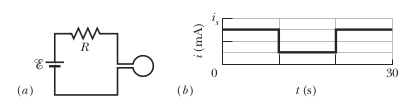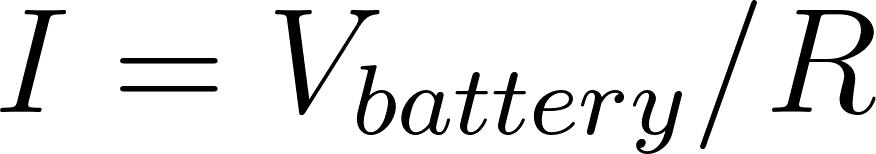×
Get Full Access to Fundamentals Of Physics - 10 Edition - Chapter 30 - Problem 6
Get Full Access to Fundamentals Of Physics - 10 Edition - Chapter 30 - Problem 6

×

# Figure 30-37a shows a circuit consisting of an idealISBN: 9781118230718 79

## Solution for problem 6 Chapter 30

Fundamentals of Physics | 10th Edition

• Textbook Solutions
• 2901 Step-by-step solutions solved by professors and subject experts
• Get 24/7 help from StudySoup virtual teaching assistantsFundamentals of Physics | 10th Edition

4 5 1 273 Reviews
24
2
Problem 6

Figure 30-37a shows a circuit consisting of an ideal battery with emf # ! 6.00 mV, a resistance R, and a small wire loop of area 5.0 cm2 . For the time interval t ! 10 s to t ! 20 s, an external magnetic field is set up throughout the loop. The field is uniform, its direction is into the page in Fig. 30-37a, and the field magnitude is given by B ! at, where B is in teslas, a is a constant, and t is in seconds. Figure 30- 37b gives the current i in the circuit before, during, and after the external field is set up. The vertical axis scale is set by is ! 2.0 mA. Find the constant a in the equation for the field magnitude.

Step-by-Step Solution:

Problem 6

Figure ‘a’ shows a circuit consisting of an ideal battery with emf = 6.00 mV, a resistance R, and a small wire loop of area 5.0 cm2 . For the time interval t =10 s to t = 20 s, an external magnetic field is set up throughout the loop. The field is uniform, its direction is into the page in Fig. a, and the field magnitude is given by B= at, where B is in teslas, a is a constant, and t is in seconds. Figure ‘b’ gives the current i in the circuit before, during, and after the external field is set up. The vertical axis scale is set by. Find the constant a in the equation for the field magnitude.Step by Step Solution

Step 1 of 3

Given at,,Step 2 of 3

Step 3 of 3

##### ISBN: 9781118230718

Unlock Textbook Solution